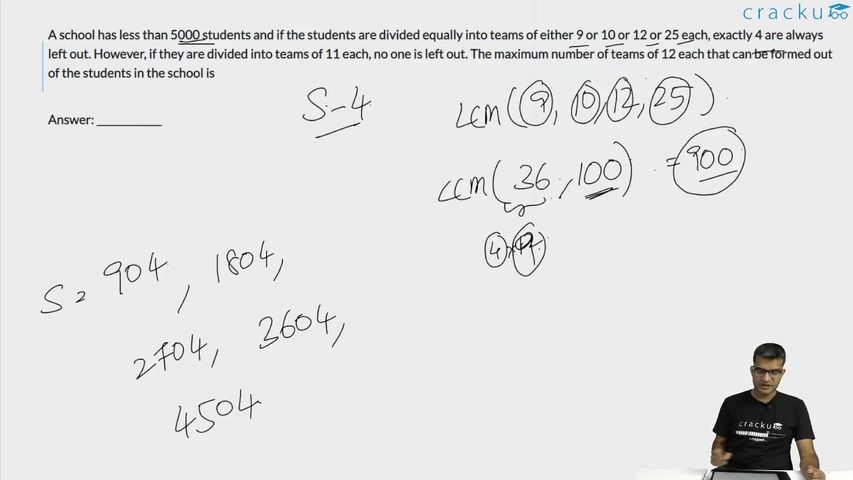Question 17

# A school has less than 5000 students and if the students are divided equally into teams of either 9 or 10 or 12 or 25 each, exactly 4 are always left out. However, if they are divided into teams of 11 each, no one is left out. The maximum number of teams of 12 each that can be formed out of the students in the school is

Solution

Since the total number of students, when divided by either 9 or 10 or 12 or 25 each, gives a remainder of 4, the number will be in the form of LCM(9,10,12,25)k + 4 = 900k + 4.

It is given that the value of 900k + 4 is less than 5000.

Also, it is given that 900k + 4 is divided by 11.

It is only possible when k = 2 and total students = 1804.

So, the number of 12 students group = 1800/12 = 150.

### View Video Solution##### Anjali Chindalia

2 months ago

How come the LCM of 10 and 25 be 100?? Pls explain. It should be 50 right ?? Kindly explain how it came 100??

##### Anubhav Guria

1 month, 4 weeks ago

Yes, it should be 50.

##### Naina Agrawal

1 month, 4 weeks ago

2*3*5*3*1*2*5= 900